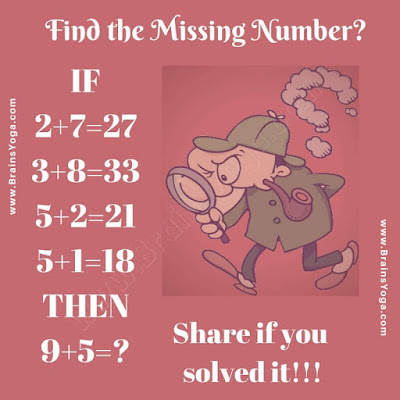Here is simple logical puzzle to test your mind. Here are some equations given which are not correct mathematically but these equation follows some logical pattern. Can you find this logical pattern and then find the value of missing number which will replace the question mark?Can you find the missing number?